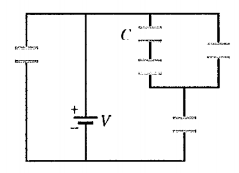# Problem: What is the equivalent capacitance of the five capacitors? All capacitors are identical and have capacitance of C = 40 nF. a. 15 nF b. 24 nF c. 25 nF d. 64 nF e. 200 nF

🤓 Based on our data, we think this question is relevant for Professor Efthimiou's class at UCF.

###### Problem Details

What is the equivalent capacitance of the five capacitors? All capacitors are identical and have capacitance of C = 40 nF.

a. 15 nF

b. 24 nF

c. 25 nF

d. 64 nF

e. 200 nF